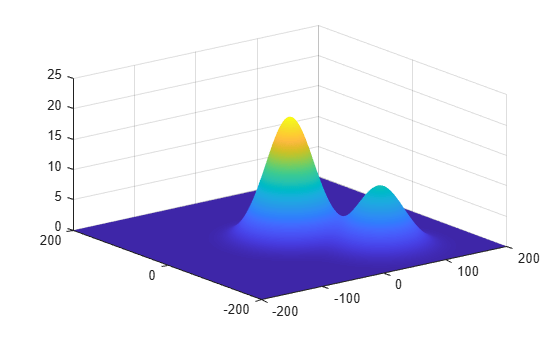# height

Height of point on surface

## Syntax

``hgt = height(surface,pt)``
``hgt = height(surface,pt,t)``

## Description

example

````hgt = height(surface,pt)` returns the height `hgt` of the point `pt` on the `surface`. This syntax applies when the surface is a `SeaSurface` object or a `LandSurface` object.```
````hgt = height(surface,pt,t)` returns the height `hgt` of the point `pt` on the `surface` at the time `t`. This syntax only applies when the `surface` is a `SeaSurface` object.```

## Examples

collapse all

Create a square sea surface area using the `seaSurface` object. Assume a moderate sea state with a wind speed of about 10 m/s, a fetch of 250 km and a length of 1.0 km. Add an Elfouhaily spectrum to the sea surface. Use the height function to determine the heights of 2 points on the map.

```scene = radarScenario(IsEarthCentered = false); rng('default')```

Add a sea surface to the scene with an Elfouhaily spectrum.

```spec = seaSpectrum(Resolution = 20); srf = seaSurface(scene,Boundary = [-500 500; -500 500], ... WindSpeed = 10,Fetch = 250000,SpectralModel = spec);```

Find the height at two points.

```P1 = [0;0]; P2 = [30;-70]; H = height(srf,[P1 P2])```
```H = 1×2 -0.9394 -0.2682 ```

Display the sea surface properties in the surface manager.

`mgr = scene.SurfaceManager`
```mgr = SurfaceManager with properties: UseOcclusion: 1 Surfaces: [1x1 radar.scenario.SeaSurface] ```
`mgr.Surfaces`
```ans = SeaSurface with properties: WindSpeed: 10 WindDirection: 0 Fetch: 250000 SpectralModel: [1x1 seaSpectrum] RadarReflectivity: [1x1 surfaceReflectivitySea] ReflectivityMap: 1 ReferenceHeight: 0 Boundary: [2x2 double] ```

Create a radar scenario. Add a 400-by-400 m area to the scenario with two simulated hiils. Find the height of two points.

```scene = radarScenario('IsEarthCentered',false); bnds = [-200 200; -200, 200]; x = -200:200; y = -200:200; [X,Y] = meshgrid(x,y); htmap = 20*exp(-X.^2/2000 - Y.^2/2000) + 10*exp(-(X-70).^2/2000 - (Y+100).^2/2000); surf(X,Y,htmap) shading interp```Find the height of the surface at two points.

```P1 = [0.0; 0.0]; % Point 1 P2 = [28.0; -40.0]; % Point 2 srf = landSurface(scene,'Terrain',htmap,'Boundary',bnds)```
```srf = LandSurface with properties: RadarReflectivity: [1x1 surfaceReflectivityLand] ReflectivityMap: 1 ReferenceHeight: 0 Boundary: [2x2 double] Terrain: [401x401 double] ```
`H = height(srf,[P1 P2])`
```H = 1×2 20.0058 6.7565 ```

Use the surface manager find the surfaces in the scenario.

`mgr = scene.SurfaceManager`
```mgr = SurfaceManager with properties: UseOcclusion: 1 Surfaces: [1x1 radar.scenario.LandSurface] ```
`mgr.Surfaces`
```ans = LandSurface with properties: RadarReflectivity: [1x1 surfaceReflectivityLand] ReflectivityMap: 1 ReferenceHeight: 0 Boundary: [2x2 double] Terrain: [401x401 double] ```

## Input Arguments

collapse all

Surface, specified as a `LandSurface` or `SeaSurface` object.

Points on surface, specified as 2-by-N matrix of real values or a 3-by-N matrix of real values, where N is the number of points.

The coordinate system of the point depends on the value of the `IsEarthCentered` property of the radar scenario object::

• `false` — Each column of the 2-by-N matrix represents the x- and y-coordinates of points in meters. Each column of the 3-by-N matrix represents the x-, y-, and z-coordinates in meters. Note that the z-coordinate is irrelevant for surface height querying.

• `true` — Each column of the 2-by-N matrix is the latitude in degrees and longitude in degrees, in the geodetic frame. Each column of the 3-by-N matrix is the latitude in degrees, longitude in degrees, and altitude in degrees, in geodetic coordinates. Note that the latitude is irrelevant for surface height querying.

Simulation time, specified as a scalar.

#### Dependencies

To enable this argument, select `surface` as a `SeaSurface` object.

Data Types: `double`

## Output Arguments

collapse all

Height of point, returned as a scalar or an N-element vector of real values, where N is the number of queried positions. Units are in meters.

## Version History

Introduced in R2022a